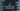# C program to print fibonacci series using recursion### C program to print Fibonacci series using recursion :

Fibonacii series is a series of numbers, where each number is equal to the sum of the previous two numbers in the series. For example , it looks like 0 1 1 2 3 5 8 13….In this example, we will learn how to print the Fibonacci series using recursion in C programming language . We will print the series till N terms . The value of N will be entered by the user and we will print the series. Let’s take a look into the _C program _:

### C Program to print fibonacci series :

``````#include <stdio.h>

void printFibonacci(int firstNo, int secondNo, int count)
{
//6
if (count > 0)
{
//7
int sum = firstNo + secondNo;
printf("%d ", sum);

//8
firstNo = secondNo;
secondNo = sum;

//9
printFibonacci(firstNo, secondNo, count - 1);
}
}

int main()
{
//1
int count;
int firstNo = 0;
int secondNo = 1;

//2
printf("Enter total numbers : ");

//3
scanf("%d", &count);

//4
printf("%d %d ", firstNo, secondNo);

//5
printFibonacci(firstNo, secondNo, count - 2);
}``````

### Explanation :

The commented numbers in the above program denotes the step number below :

1. Create three different variables count, firstNo and secondNo. count will hold the total numbers in the series we are going to print, firstNo holds the first number in the series i.e. 0 and secondNo holds the second number in the series i.e. 1.
2. Ask the user to enter the total numbers to print for the series.
3. Store the number count in variable count.
4. Start printing the series. First print firstNo and secondNo.
5. Call the function printFibonacci to print other numbers.
6. We will decreate the count each time. count = n means we have n numbers to print. So, we will not do anything if count is 0.
7. Create the sum of firstNo and secondNo and print out the result.
8. Store the value of secondNo on firstNo and store the value of sum on secondNo.
9. Now , call the method printFibonacci again with the new firstNo, secondNo and decreasing count by 1.

### Sample Output :

``````Enter total numbers : 7
0 1 1 2 3 5 8

Enter total numbers : 20
0 1 1 2 3 5 8 13 21 34 55 89 144 233 377 610 987 1597 2584 4181``````